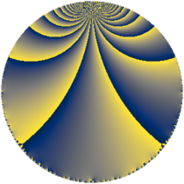# Properties

 Label 1849.4.eLevel $1849$ Weight $4$ Character orbit 1849.e Rep. character $\chi_{1849}(78,\cdot)$ Character field $\Q(\zeta_{7})$ Dimension $2586$ Sturm bound $630$

# Related objects

## Defining parameters

 Level: $$N$$ $$=$$ $$1849 = 43^{2}$$ Weight: $$k$$ $$=$$ $$4$$ Character orbit: $$[\chi]$$ $$=$$ 1849.e (of order $$7$$ and degree $$6$$) Character conductor: $$\operatorname{cond}(\chi)$$ $$=$$ $$43$$ Character field: $$\Q(\zeta_{7})$$ Sturm bound: $$630$$

## Dimensions

The following table gives the dimensions of various subspaces of $$M_{4}(1849, [\chi])$$.

Total New Old
Modular forms 2970 2826 144
Cusp forms 2706 2586 120
Eisenstein series 264 240 24

## Trace form

 $$2586q + 9q^{2} + 3q^{3} - 1649q^{4} + 23q^{5} - 16q^{6} - 96q^{7} - 61q^{8} - 3414q^{9} + O(q^{10})$$ $$2586q + 9q^{2} + 3q^{3} - 1649q^{4} + 23q^{5} - 16q^{6} - 96q^{7} - 61q^{8} - 3414q^{9} + 61q^{10} - 73q^{11} - 33q^{12} - 103q^{13} + 299q^{14} - 109q^{15} - 6089q^{16} - 189q^{17} + 414q^{18} - 284q^{19} + 363q^{20} + 88q^{21} - 421q^{22} - 257q^{23} + 937q^{24} - 9138q^{25} - 139q^{26} + 27q^{27} - 29q^{28} + 367q^{29} - 1244q^{30} + 269q^{31} - 435q^{32} + 2594q^{33} + 583q^{34} + 902q^{35} + 71024q^{36} - 1020q^{37} - 1251q^{38} + 1571q^{39} - 1263q^{40} - 373q^{41} + 1830q^{42} - 7724q^{44} - 1019q^{45} + 2786q^{46} + 411q^{47} + 95q^{48} + 91838q^{49} + 282q^{50} - 1524q^{51} + 1575q^{52} + 1389q^{53} + 4231q^{54} + 1735q^{55} + 1895q^{56} - 1055q^{57} - 335q^{58} - 591q^{59} + 591q^{60} + 339q^{61} - 923q^{62} + 702q^{63} - 13653q^{64} - 2463q^{65} - 747q^{66} + 83q^{67} - 3032q^{68} - 2711q^{69} + 7698q^{70} + 2431q^{71} + 4340q^{72} + 2157q^{73} + 1196q^{74} + 242q^{75} + 4272q^{76} + 3962q^{77} + 2860q^{78} + 468q^{79} - 11618q^{80} - 34173q^{81} - 4023q^{82} + 2458q^{83} - 3852q^{84} + 3446q^{85} - 17106q^{87} - 9957q^{88} + 5811q^{89} + 2688q^{90} + 760q^{91} + 376q^{92} - 3994q^{93} - 6057q^{94} + 211q^{95} + 1022q^{96} + 5989q^{97} + 9041q^{98} + 2462q^{99} + O(q^{100})$$

## Decomposition of $$S_{4}^{\mathrm{new}}(1849, [\chi])$$ into newform subspaces

The newforms in this space have not yet been added to the LMFDB.

## Decomposition of $$S_{4}^{\mathrm{old}}(1849, [\chi])$$ into lower level spaces

$$S_{4}^{\mathrm{old}}(1849, [\chi]) \cong$$ $$S_{4}^{\mathrm{new}}(43, [\chi])$$$$^{\oplus 2}$$